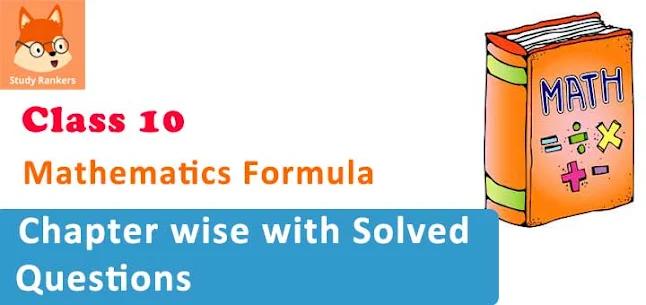# Important CBSE Mathematics Formula Booklet for Class 10 - Free PDF Download

CBSE Maths formula for Class 10 is very important while solving the questions from mathematics textbooks. In this page, our experts have prepared the important mathematics formula from the NCERT textbook of Class 10 Maths. Students can download these Maths Formula and use it for their future references. By learning these mathematics formula, students would be able to:

• Solve questions easily by applying the formula.
• Save time and doing revision.
• Get good marks in the examination. Very helpful in doing quick revision.
• Memorising these, students can get themselves prepared for board and other competitive examinations.These class 10 maths formula are prepared chapterwise. Our expert faculty has also provided the solved examples of the formula. This makes the students easier to understand the concepts and apply formula accordingly.

 Title Maths Formula for Class 10 Board CBSE and State Boards Related Study materials

By studying the given maths formula for class 10, students can secure good marks by taking examination. It will boost their confidence. It also help them in achieving greater success.

## Maths Formula for Class 10| Chapterwise

• Chapter 1 – Real Numbers Formulas
• Chapter 2 – Polynomials Formulas
• Chapter 3 – Pair of Linear Equations in Two Variables Formulas
• Chapter 4 – Quadratic Equations Formulas
• Chapter 5 – Arithmetic Progressions Formulas
• Chapter 6 – Triangles Formulas
• Chapter 7 – Coordinate Geometry Formulas
• Chapter 8 – Introduction to Trigonometry Formulas
• Chapter 10 – Circles Formulas
• Chapter 12 – Areas Related to Circles Formulas
• Chapter 13 – Surface Areas and Volumes Formulas
• Chapter 14 – Statistics Formulas
• Chapter 15 – Probability Formulas

Students can also download the pdf of maths formula. Above formula are chaterwise with solved questions based on the formula. NCERT Solutions for Class 10 Maths can also be studied by students to have an idea about implementation of formula in the questions. Illustrative and Practical examples provided with class 10 maths formulas give an exact idea to the children.